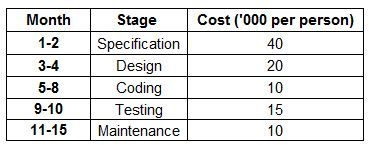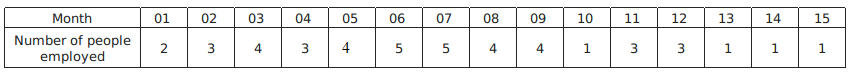### CAT 1996 Question Paper Question 57

Instructions

Answer the questions based on the following information. Mulayam Software Co., before selling a package to its clients, follows the given schedule.The number of people employed in each month is:Question 57

# With reference to the above question, what is the cost incurred in the new ‘coding’ stage? (Under the new technique, 4 people work in the sixth month and 5 in the eighth.)

Solution

As coding is done in 6-8 months,
So total person employed = 4+5+5 = 14
cost per person for 6-8 month = 10000
Total cost will be = $$(14 \times 10000)$$ = 140,000

• All Quant CAT Formulas and shortcuts PDF
• 30+ CAT previous papers with solutions PDF